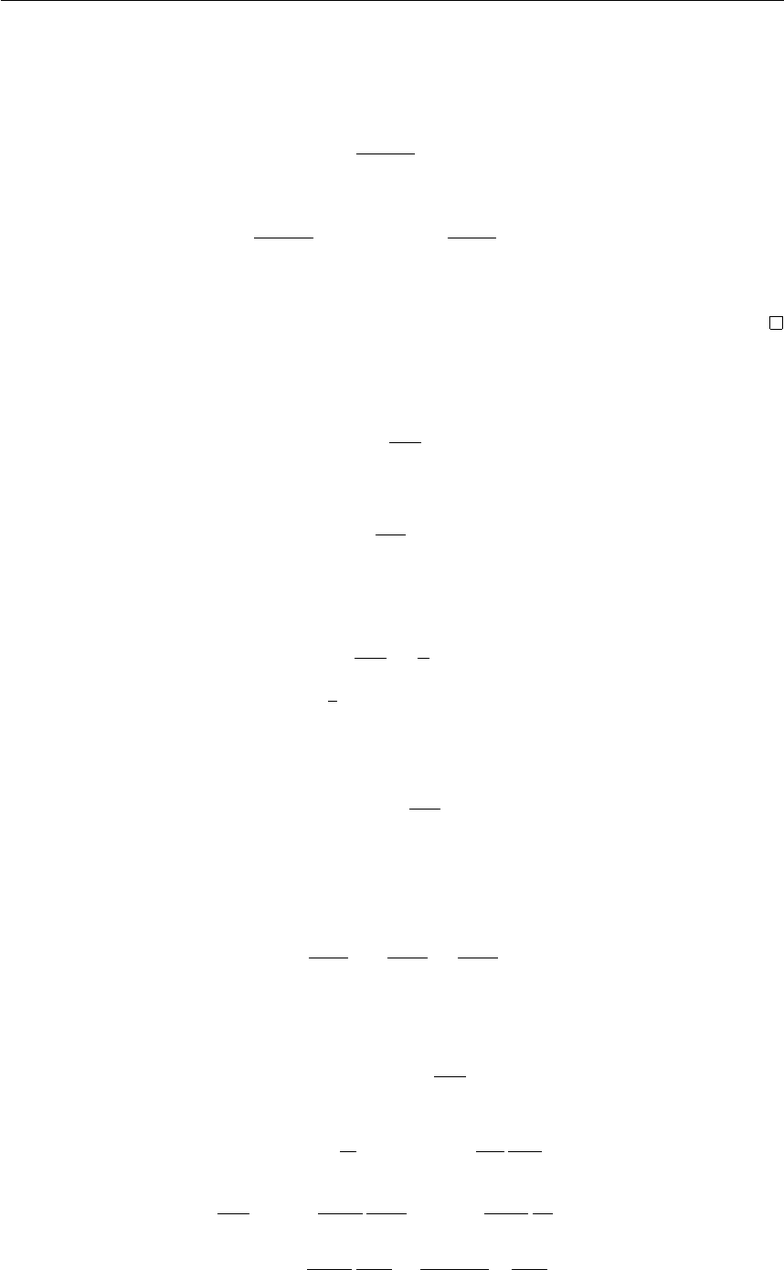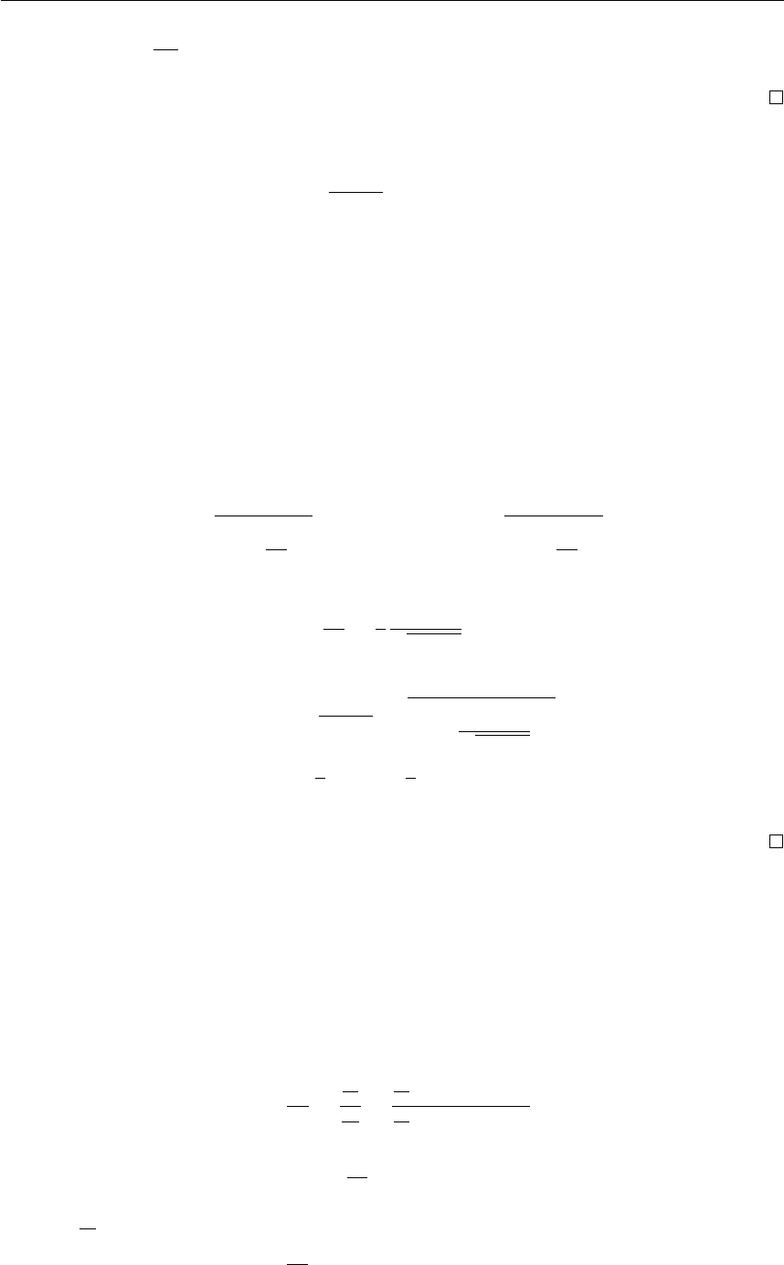Study Guides (380,000)
CA (150,000)
UW (6,000)
MATH (500)
MATH128 (60)
Final

# MATH128 Study Guide - Final Guide: Ibm System P, Alternating Series, Conditional Convergence

Department
Mathematics
Course Code
MATH128
Professor
Clinton Loo
Study Guide
Final

This preview shows pages 1-2. to view the full 7 pages of the document.Calculus II 1
2010-06-14
Calculus II : Practice ﬁnal (Calculators and textbooks are NOT allowed during the exam. )
1. Evaluate the integral
Z3w1
w+ 2 dw.
Z3w1
w+ 2 dw =Z37
w+ 2dw
= 3w7 ln |w+ 2|+C
2. Find the value of pfor which the series is convergent
X
n=1
ln n
np.
If p0, then
lim
n→∞
ln p
np=
so by the divergent test, this series is divergent for p0.
If p= 1, then
ln n
n1
n
for any positive integer n. Since P
n=1 1
nis divergent, by the comparison test, this series is also
divergent.
For p > 0and p6= 1, let
f(x) = ln x
xp
for x1. Then
f(x)is positive for x > 1and continuous on [1,)
since
f0(x) = 1
xp+1 pln x
xp+1 =1
xp+1 (1 pln x)
so f0(x)<0for x>e1/p. So f(x)is decreasing for (e1/p,).
for each integer n1,
f(n) = ln n
np
By the integral test, this series is convergent if and only if R
1f(x)dx is convergent. Use
integration by part, let u= ln xand dv =1
xpdx (then v=1
1p
1
xp1if p6= 1)
Zt
1
ln x
xpdx =1
1p
ln x
xp1t
1Zt
1
1
1p
1
xpdx
=1
1p
ln t
tp11
(1 p)21
tp11

Only pages 1-2 are available for preview. Some parts have been intentionally blurred.Calculus II 2
Then the limit R
1
ln x
xpdx is convergent whenever p1>0.
Therefore, this series is convergent if and only if p > 1.
3. Find the surface area of the surface obtained by rotating
y=p7x2,0x1
Surface area S:
S=Z2πy ds
S=Z2πx ds
where
ds =s1 + dx
dy 2
dy or ds =s1 + dy
dx2
dx.
This case, dy
dx =1
22x
7x2
so
S= 2πZ1
0p7x2·s1 + x
7x22
dx
= 2πZ1
0
7dx = 27π
4.
(a) Sketch the curve
r= cos 2θ.
Four-leaved rose. (p. 643)
(b) Find the points on the curve r= cos 2θwhere the tangent line is horizontal or vertical.
dy
dx =
dy
dx
=
dr
sin θ+rcos θ
dr
cos θrsin θ
dr
=2 sin 2θ
dy
= 0
dy
=2 sin 2θsin θ+ cos 2θcos θ= 0
⇒ −4 sin2θcos θ+ (1 + 2 cos2θ) cos θ= 0